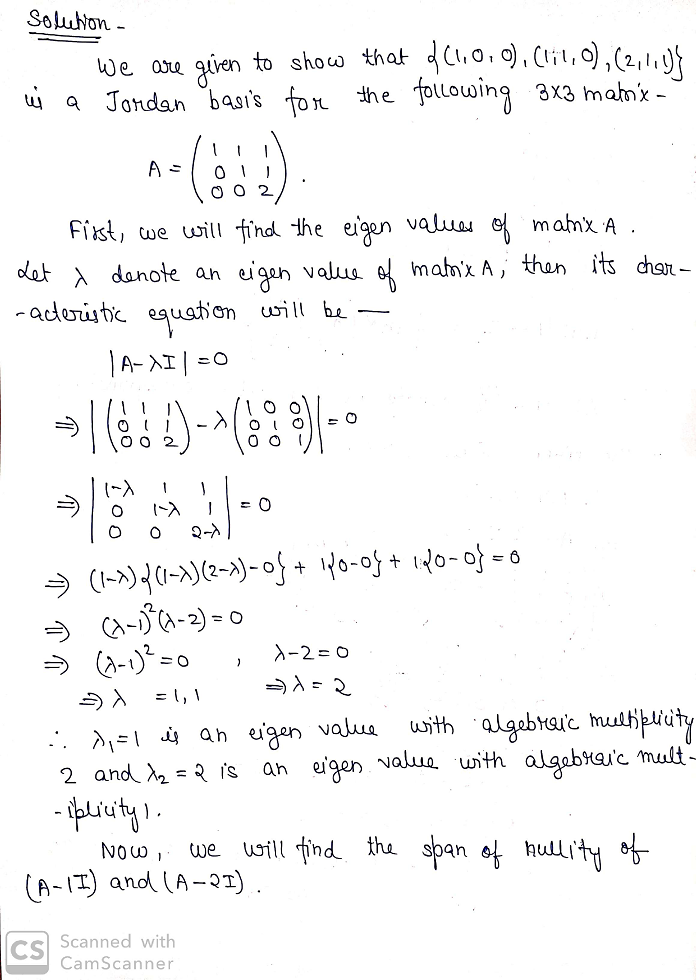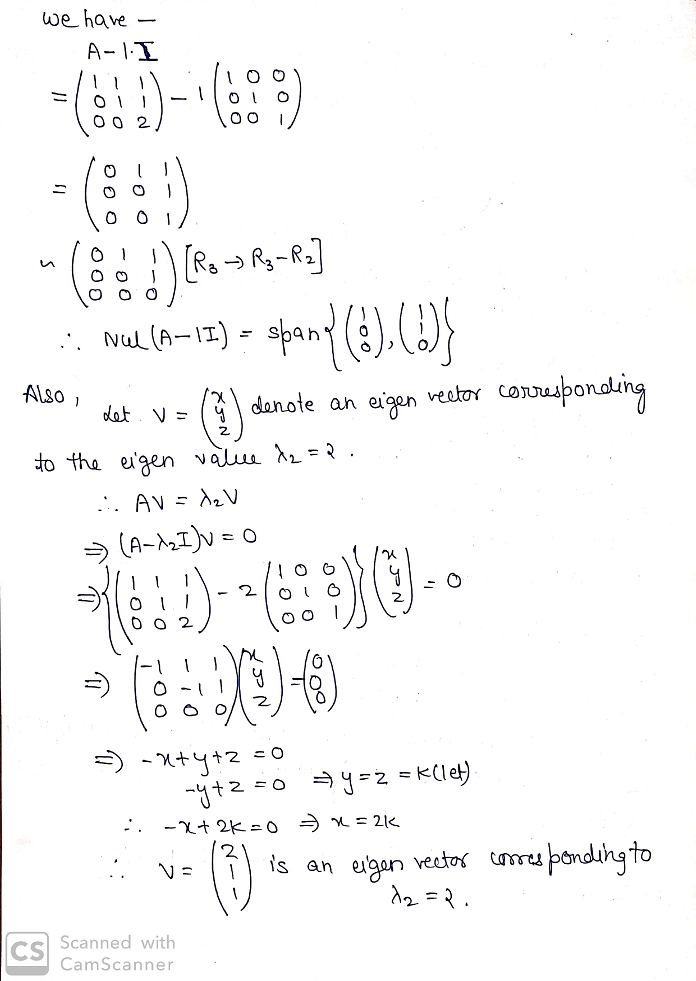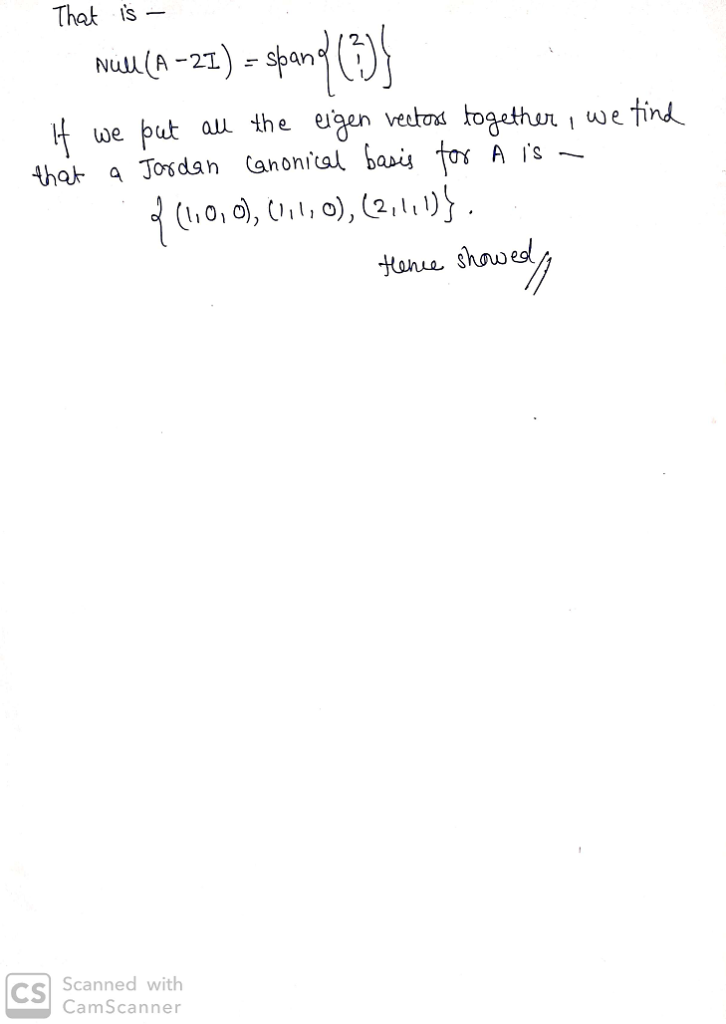Homework Help Question & Answers

Hello, my problem is the following: Check that (1,0,0),(1,1,0),(2,1,1) is a Jordan basis for (1,1...

Hello, my problem is the following:

Check that (1,0,0),(1,1,0),(2,1,1) is a Jordan basis for (1,1,1 (first row from top) 0,1,1 (second row from top) 0,0,2(third row from top)). Can somebody give me a step-by-step explanation for this?

The solution is attached herewith.

Add Answer of: Hello, my problem is the following: Check that (1,0,0),(1,1,0),(2,1,1) is a Jordan basis for (1,1...
More Homework Help Questions Additional questions in this topic.

• 15. Let F(z,y)- F dr where C is any positively-oriented Jordan curve that encloses the origin Evaluate 15. Let F(z,y)- F dr where C is any positively-oriented Jordan curve that encloses the o...

Need Online Homework Help?

Get FREE EXPERT Answers
WITHIN MINUTES
Related Questions